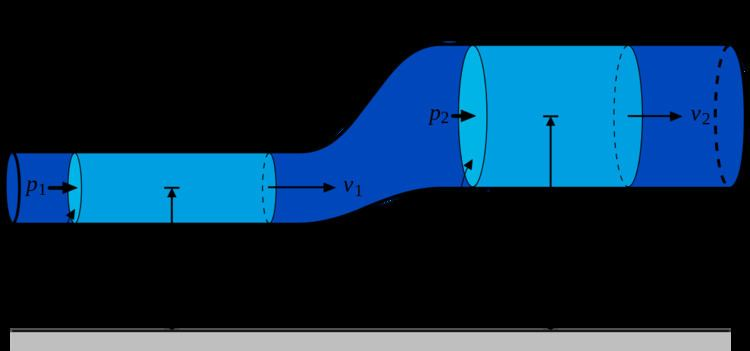# Fluid

Updated on
Edit
Like
CommentIn physics, a fluid is a substance that continually deforms (flows) under an applied shear stress. Fluids are a subset of the phases of matter and include liquids, gases, plasmas, and to some extent, plastic solids. Fluids are substances that have zero shear modulus, or, in simpler terms, a fluid is a substance which cannot resist any shear force applied to it.

## Contents

Although the term "fluid" includes both the liquid and gas phases, in common usage, "fluid" is often used as a synonym for "liquid", with no implication that gas could also be present. For example, "brake fluid" is hydraulic oil and will not perform its required incompressible function if there is gas in it. This colloquial usage of the term is also common in medicine and in nutrition ("take plenty of fluids").

Liquids form a free surface (that is, a surface not created by the container) while gases do not. The distinction between solids and fluid is not entirely obvious. The distinction is made by evaluating the viscosity of the substance. Silly Putty can be considered to behave like a solid or a fluid, depending on the time period over which it is observed. It is best described as a viscoelastic fluid. There are many examples of substances proving difficult to classify. A particularly interesting one is pitch, as demonstrated in the pitch drop experiment currently running at the University of Queensland.

## Physics

Fluids display properties such as:

• not resisting deformation, or resisting it only slightly (viscosity), and
• the ability to flow (also described as the ability to take on the shape of the container).This also means that all liquids have the property of fluidity.
• These properties are typically a function of their inability to support a shear stress in static equilibrium.

Solids can be subjected to shear stresses, and to normal stresses—both compressive and tensile. In contrast, ideal fluids can only be subjected to normal, compressive stress which is called pressure. Real fluids display viscosity and so are capable of being subjected to low levels of shear stress.

## Modelling

In a solid, shear stress is a function of strain, but in a fluid, shear stress is a function of strain rate. A consequence of this behavior is Pascal's law which describes the role of pressure in characterizing a fluid's state.

Depending on the relationship between shear stress, and the rate of strain and its derivatives, fluids can be characterized as one of the following:

• Newtonian fluids: where stress is directly proportional to rate of strain
• Non-Newtonian fluids: where stress is not proportional to rate of strain, its higher powers and derivatives.
• The behavior of fluids can be described by the Navier–Stokes equations—a set of partial differential equations which are based on:

• continuity (conservation of mass),
• conservation of linear momentum,
• conservation of angular momentum,
• conservation of energy.
• The study of fluids is fluid mechanics, which is subdivided into fluid dynamics and fluid statics depending on whether the fluid is in motion.

Fluid Wikipedia